## Python Basics Day 1 Starter

### 1.1 Complete activities below from Home to Python Variables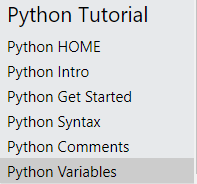1. Write a program that will ask the user for three integer numbers and then add these numbers
together before displaying the answer. The input and output should be user friendly.

## Python Basics 23 Nov 2021

### 1.1 Complete activities below from Data Types to Strings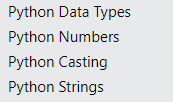## Python Basics Day 07 Sep 2021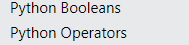## Python Basics 10 Sep 2021

4.1.9 Learning Objectives ( thinking ahead )

Identify the inputs and outputs required in a solution.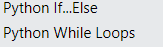### Programming Activity

The program below will always prompt for 2 numbers and add these together. You have  been asked to extend the program below to first prompt the use for how many numbers they wish to add, if they answer 3 for example then prompt for 3 numbers and add them together and print out the result.!!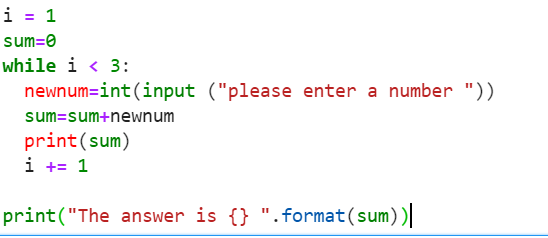## Python Basics 14 Sep 2021

4.1.5 - Identify the decisions required for the solution to a specified problem. (Different actions are taken based on conditions.)

Identify the inputs and outputs required in a solution.

### Programming Activity

Today we are going to make an interactive guessing game in Python.

This is going to be a simple guessing game where the computer will generate a random number between 1 to 10, and the user has to guess it in 5 attempts.

Based on the user’s guess computer will give various hints if the number is high or low. When the user guess matches the number computer will print the answer along with the number of attempts.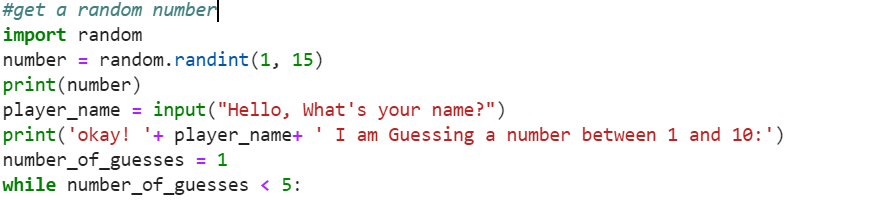Game Building https://replit.com/curriculum/Build-a-Simple-Game-with-Python

## 1.3 Using the table below try and complete 1 - 4

Try to complete the following exercises; the table above will help you:

1. Write a program that will ask the user for three integer numbers and then add these numbers
together before displaying the answer. The input and output should be user friendly.

2. Write a program that will ask the user for four integer numbers and then add them together
and then divide that total by 4 to show the average. The input and output should be user
friendly.

3. Write a program that will ask for the total bill at the restaurant and then the number of diners
who ate that meal. It will then work out how much each person should pay for an equal share
of the meal.

4. Write a program that will ask the user the height and then the width of a rectangle and which
will then show the area of the rectangle.

## Python Basics  21 Sep Input Statements and if Statements

### Part 1 Input Statements and If Statments3 Challenges

4.1.5 - Identify the decisions required for the solution to a specified problem. (Different actions are taken based on conditions.)

#### WALT: Use input statements in Python

Coding challenge 1  Complete the program below, but change it to be an English to Thai Translator, if wish you can change the input list from animals to another subject example food.

Extra Challenge :  Can you extend to use a loop so that the translator will continue to work until the user enters 99 to stop the program?

Coding challenge 2: Write a program that asks the user to enter how many children are in their class and how many exercise books each child has. The program should then output how many exercise books there are altogether.

Coding challenge 3: Write a program which asks the user their name and how many days until their next birthday. The program should then output a personalized sentence with a rough approximation of the number of seconds until their next birthday.

### Lesson 1.2 Conditional Statements

Complete the program below

Program Challenge in Python

Create Program "Find The Skeleton"

Scenario 4 rooms and 2 attempts to find the skeleton

If the user selects the wrong room, inform them how many tries left and also give them a clue.

## Lesson 2 Arithmetic Operators

Try to complete the following exercises; the table above will help you:

1. Write a program that will ask the user for three integer numbers and then add these numbers
together before displaying the answer. The input and output should be user friendly.

2. Write a program that will ask the user for four integer numbers and then add them together
and then divide that total by 4 to show the average. The input and output should be user
friendly.

3. Write a program that will ask for the total bill at the restaurant and then the number of diners
who ate that meal. It will then work out how much each person should pay for an equal share
of the meal.

4. Write a program that will ask the user the height and then the width of a rectangle and which
will then show the area of the rectangle.

5. Alter the previous program so that it will ask for the height, width and also the depth of a box
and then show the total volume of that box.

6. Write a program which will ask for the height, width and depth of a small box and then the
height, width and depth of a larger box. Work out how much space is remaining around the
small box when it is placed inside the bigger box.

7. Write a program that will ask the user for how many sweets are in the bag. It should also ask
how many people the sweets should be shared between. Use the whole number division to
work out how many sweets each person should have and use the remainder division to find out
how many sweets would be left remaining. For example if there are 14 sweets in the bag and
this was to be divided between 3 people then the programme should display “There will be 4
sweets each and there would be 2 left over.”

4.1.5 - Identify the decisions required for the solution to a specified problem. (Different actions are taken based on conditions.)

# Description: This program prints a message

# stored in a variable 10 times

message1 = "Outside of loop."

message2 = "I am in a while loop."

counter = 0

print(message1)

while(counter < 10):

print(message2)

counter = counter + 1

print(message1)

### Presentation with Working Code

We have looked at 2 loop structures the For and the While Loop.

Create a presentation that describes the difference between thee 2 loop structures.

Maximum of 5 slides

You also need 2 working python programs that demonstrate the difference between these 2 loops

## Activity Text Based Adventure Game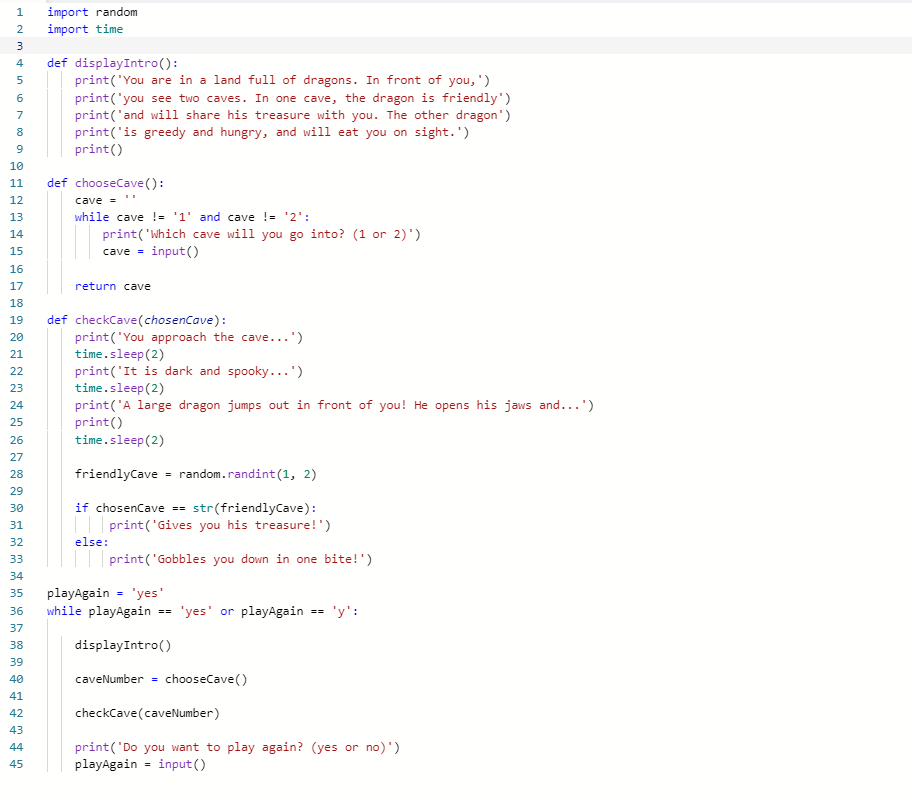Independent work:

Enhance the example code to extend the number of caves to 5 and make changes to the text.. you can choose a completley different text adventurev game

Scroll to Top